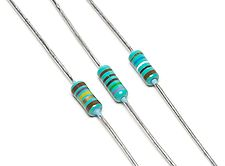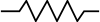A vehicle going in very high speed can avoid mishap or control its flow by applying brakes or speed breakers.While applying brakes, they stop or prevent the wheels from moving. Thus a vehicle can be saved from damage by applying brakes.

Like the function of brakes in vehicle, resistors are to control to flow or speed of electrical current to save the device from damage.

Resistance of an object is a measure for impeding or controlling the flow of electrical current.
Resistor is a passive device that opposes the circulation of current and deveops a voltage across its terminals . By Ohm's law, Voltage is proportional to electrical current.##### V = RI

where R is resistance of an object and measured in Ohm(Ω). Computation of resistance of a material or conductor requires some parameters of that material. Resistance can be calculated from the following formula

##### R = l ρ / A

where l is length of the material measured in meters and A is area of cross section in meters and Resistivity(ρ),which is how much material's capability to resist electric current, is measured in Ohm meter.Most commonly usages of SI unit of resistance are milliohm(10-3 Ω),kiloohm(103 Ω ) and megaohm(106 Ω).

The symbol for resistor :Reciprocal of resistance is electrical conductance and it is measured in siemans (S).Practical resistors are manufactured from many compounds and films.